# Perimeter of rhombus

The area of the rhombus is 32 cm2. One side of it measures 10 cm. The height on the other side measures 5 cm. What is the circumference of this rhombus?

o =  32.8 cm

### Step-by-step explanation: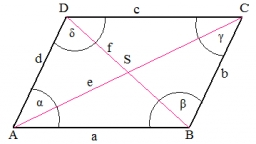Did you find an error or inaccuracy? Feel free to write us. Thank you!#### You need to know the following knowledge to solve this word math problem:

We encourage you to watch this tutorial video on this math problem:

## Related math problems and questions:

• Rhombus A2p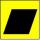Rhombus area is 13 cm2 and its height is 5cm long . Determine a perimeter of this rhombus.
• PrismCalculate the surface area and volume of a prism with a body height h = 10 cm, and its base has the shape of a rhomboid with sides a = 5.8 cm, b = 3 cm, and the distance of its two longer sides is w = 2.4 cm.
• RhombusFind the length of the other diagonal and area of the rhombus. The perimeter of a rhombus is 40 cm and one of the diagonals is of length 10 cm.
• RhombusIf we increase the rhombus side by 2.4 cm; its perimeter is 20 cm. How long was the side of the rhombus?
• RhumblineFind circumference and area of the rhumbline ABCD if the short side AD of which has a length of 5 cm, and the heel of the height from D leading to the AB side divides the AB side into two sections of 3 cm and 4 cm.
• Silo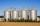Outer perimeter of silo is 32 m. Concrete wall is 35 cm thick. What is diameter and area of inside floor of silo?
• RhombusPQRS is a rhombus. Given that PQ=3 cm & height of rhombus is given 2 cm. Calculate its area.
• Parallerogram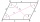In parallelogram ABCD is a = 2.5 cm and height to side a is 7.2 cm and height to side b is 4 cm. Find the side length b.
• PrismThe base of the prism is a rhombus with a side 30 cm and a height 27 cm long. The height of the prism is 180% longer than the side length of the rhombus. Calculate the volume of the prism.
• Two patches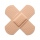Peter taped the wound with two rectangular patches (one over the other to form the letter X). The area sealed with both patches at the same time had a content of 40cm2 and a circumference of 30cm. One of the patches was 8cm wide. What was the width of the
• Rhombus 4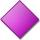The circumference of the rhombus is 44 cm, its height is 89 mm long. Calculate its content area.
• Four prismsQuestion No. 1: The prism has the dimensions a = 2.5 cm, b = 100 mm, c = 12 cm. What is its volume? a) 3000 cm2 b) 300 cm2 c) 3000 cm3 d) 300 cm3 Question No.2: The prism base is a rhombus with a side length of 30 cm and a height of 27 cm. The height of t
• Do you solve this?Determine area S of rectangle and length of its sides if its perimeter is 102 cm.
• Rectangles - sidesOne side of the rectangle is 10 cm longer than second. Shortens longer side by 6 cm and extend shorter by 14 cm increases the area of the rectangle by 130 cm2. What are the dimensions of the original rectangle?
• Math heart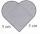Stylized heart shape created from a square with side 5 cm and two semicircles over his sides. Calculate the content area and its circumference.
• Calculate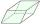Calculate the content cm2 diamond with side length 9.8 cm and a height on the side of 5 cm.
• The rectangleThe rectangle has a circumference of 32 m. One side is 2 m longer than the other side. Calculate the side lengths of this rectangle.